Next: Expression for Momentum Density Up: Basic Equation of Fluid Previous: Lagrangian and Euler Equations   Contents

# Continuity Equation

Another basic equation comes from the mass conservation. This is often called the continuity equation, which relates the change of the volume to its density. Consider a fluid element whose volume is equal to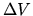. The mass contained in the volume is constant. Thus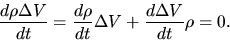(A.8)

The variation of the volume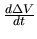is rewritten as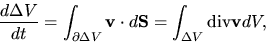(A.9)

where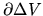represents the surface of the fluid element. From equations (A.8) and (A.9), we obtain the mass continuity equation for Lagrangian time derivative as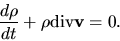(A.10)

Using equation (A.6) this is rewritten to Eulerian form as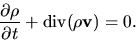(A.11)

Basic equations using the Lagrangian derivative are equations (A.3) and (A.10), while those of the Euler derivative are equations (A.7) and (A.11).

SubsectionsNext: Expression for Momentum Density Up: Basic Equation of Fluid Previous: Lagrangian and Euler Equations   Contents
Kohji Tomisaka 2007-07-08